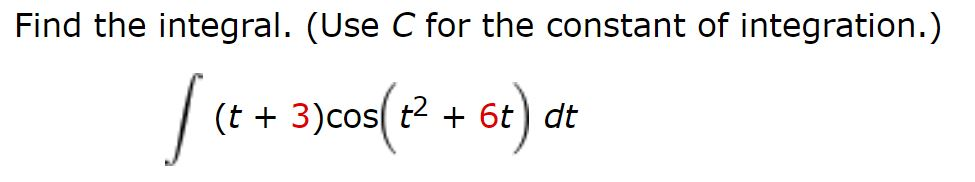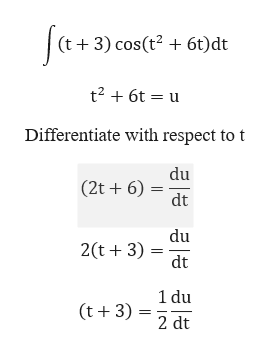# Find the integral. (Use C for the constant of integration.)[(++ 3)cos( 2 + 6t) at(t + 3)cos( t26t) dt

Question
14 viewshelp_outlineImage TranscriptioncloseFind the integral. (Use C for the constant of integration.) [(++ 3)cos( 2 + 6t) at (t + 3)cos( t2 6t) dt fullscreen
check_circle

Step 1

To find the integral

Step 2

To begin with the integration, we use the substitution method.help_outlineImage Transcriptionclose|(t + 3) cos(t? + 6t)dt t2 + 6t = u Differentiate with respect to t du (2t + 6) dt du 2(t + 3) dt 1 du (t + 3) : 2 dt %3D fullscreen
Step 3

Substitute in the...

### Want to see the full answer?

See Solution

#### Want to see this answer and more?

Solutions are written by subject experts who are available 24/7. Questions are typically answered within 1 hour.*

See Solution
*Response times may vary by subject and question.
Tagged in

### Calculus